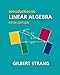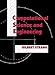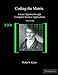Full Title Applied Linear Algebra

Short Title Applied Linear Algebra

Code MATH09003
 Level 09
 Credit 05

Author Creedon, Leo
Department Mech. and Electronic Eng.

Subject Area Mathematics
Attendence N/A%

Description

The subject covers the linear algebra required for post-graduate engineering courses.  The learner will gain the expertise to interpret the linear algebra models used in the engineering literature. It will also enable learners to model problems using linear algebra methods.

Indicative Syllabus

Review solutions of linear equations using Gauss Elimination / Back-substitution / Elementary Row operations / pivots / REF / Gauss-Jordan elimination / RREF

- Consistency and systems with unique/multiple/zero solutions

- (Geometry of Solutions) (solutions of equations as points, lines and planes)

- Complete solution to AX = B (column space containing b, rank and nullspace of A and special solutions to AX = 0 from row reduced R)

Matrix Algebra

Review addition and multiplication of matrices and vectors and their transposes.

- Inverse matrices. Calculation via Gauss Elimination. Invertibility.

- Block multiplication

3D Vectors/Rotation

- Inner product, length, orthogonality cosine rule/angles, cross product

- Rotation matrices, Euler angles and non-commutativity

- Projection, reflection, scaling

- Lines / planes in 3 dimensions

- Linear transformations and change of basis (dual basis)

Vector spaces and subspaces

- Basis and dimension / linear independence and span

- Four fundamental subspaces ( row, column, right-left nullspaces, and their bases)

Least squares solutions, overdetermined systems (closest line by understanding projections)

Orthogonalization by Gram-Schmidt (factorization into A = QR)

Orthonormal matrices  (rotation matrices as)

Determinants / as volume / cofactor formula / applications to inverses / calculation via Gauss elimination

Eigenvalues / SVD

- Eigenvalues and eigenvectors (diagonalizing and computing powers of matrices)

- Matrix exponentials. Solving difference and differential equations

- Symmetric matrices and positive definite matrices (real eigenvalues and orthogonal eigenvectors, tests for x'Ax > 0, applications) A=QLQT

- Singular Value Decomposition (SVD)

- The Moore-Penrose Pseudoinverse. Least solution underdetermined system.

LU Factorisation

Skew-symmetric matrices / skew matrices

Fourier matrices / fast Fourier transform

Graphs and networks

Kronecker product

Learning Outcomes
On completion of this module the learner will/should be able to
1. Solve systems of linear equations and analyse the solutions.

2. Analyse affine transformations in three dimensions.

3. Interpret the linear algebra in state of the art research publications and reproduce findings.

4. Explain the use of vector spaces in analysing solutions to systems of equations.

5. Use projections to find the least squares solution of overdetermined systems.

6. Decompose matrices into their singular value decompositions and interpret.

7. Apply matrices to the Fourier transform, graphs and networks.

Assessment Strategies

A terminal exam and continuous assessment will be used to assess the module.

To reinforce the theoretical principles covered in lectures, learners will participate in project work.

The learner will complete a final exam at the end of the semester.

The learner is required to pass both the projects and terminal examination element of this module.

Module Dependencies
Pre Requisite Modules
Co Requisite Modules
Incompatible Modules

Coursework Assessment Breakdown %
Course Work / Continuous Assessment 60 %
End of Semester / Year Formal Examination 40 %

Coursework Assessment Breakdown

Description Outcome Assessed % of Total Assessment Week
CA 1 1,2,4 30 Week 6
Project 1,2,3,4,5,6,7 30 Week 12

End Exam Assessment Breakdown

Description Outcome Assessed % of Total Assessment Week
Terminal Exam 1,2,3,4,5,6,7 40 End of Semester

Type Location Description Hours Frequency Avg Weekly Workload
Lecture Lecture Theatre Lecture 2 Weekly 2.00
Laboratory Practical Computer Laboratory Laboratory Practical 2 Fortnightly 1.00
Independent Learning Not Specified Independent Learning 7 Weekly 7.00

Total Average Weekly Learner Workload 3.00 Hours

Type Location Description Hours Frequency Avg Weekly Workload

Total Average Weekly Learner Workload 0.00 Hours

Type Location Description Hours Frequency Avg Weekly Workload

Total Average Weekly Learner Workload 0.00 Hours

Type Location Description Hours Frequency Avg Weekly Workload
Lecture Online Theory Lecture 1 Weekly 1.00
Independent Learning Not Specified Independent Learning 8.5 Weekly 8.50
Laboratory Practical Online Online lab 0.5 Weekly 0.50

Total Average Weekly Learner Workload 1.50 Hours

Resources
Book Resources

Other Resources

x

Url Resources

x

 Book Cover Book DetailsGilbert Strang 2016 Introduction to Linear Algebra Wellesley-Cambridge Press ISBN-10 0980232775 ISBN-13 9780980232776Gilbert Strang 2007 Computational Science and Engineering Wellesley-Cambridge Press ISBN-10 0961408812 ISBN-13 9780961408817Philip N Klein 2013 Coding the Matrix: Linear Algebra through Applications to Computer Science Newtonian Press ISBN-10 0615880991 ISBN-13 9780615880990